• 求解函数最小值的自适应遗传算法 （Matlab程序）
• 求下列二元函数的最大值，f(x1,x2)=x1^2+x2^2,x1与x2的取值区间为{0,1,2，...，7}
• 本代码主要利用MATLAB工具实现MATLAB——求解无约束非线性函数最小值，简单明了，易于理解
• 布谷鸟求解函数最小值，这是布谷鸟算法与莱维飞行的详细讲解；带注释的布谷鸟算法，清晰易懂，简洁好用，可以更改适应度函数应用不同的场景。
• 布谷鸟求解函数最小值，这是布谷鸟算法与莱维飞行的详细讲解（https://blog.csdn.net/zyqblog/article/details/80905019） 。
• 实验五 用matlab求二元函数的极值 1计算二元函数的极值 对于二元函数的极值问题,根据二元函数极值的必要和充分条件,可分为以下几个步骤: z?f(x,y. 定义二元函数步骤1.f(x,y?0,f(x,y?0yx,得到驻点. 步骤2.求解方程组...
• 一、传统遗传算法求解函数最小值 ①对于函数 其最小值位于时，最小值为0 matlab多次结果如下： 从上图能够看到传统能够得到最终结果，但是稳定性较差，不易得到较好的结果 ②对函数做平移，即可得到 其...
一、传统遗传算法求解函数最小值
①对于函数

其最小值位于时，最小值为0
matlab多次结果如下：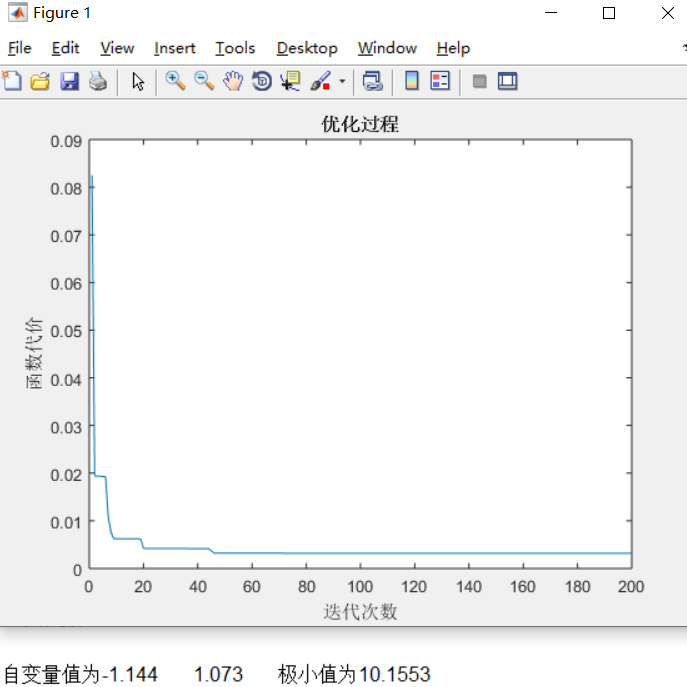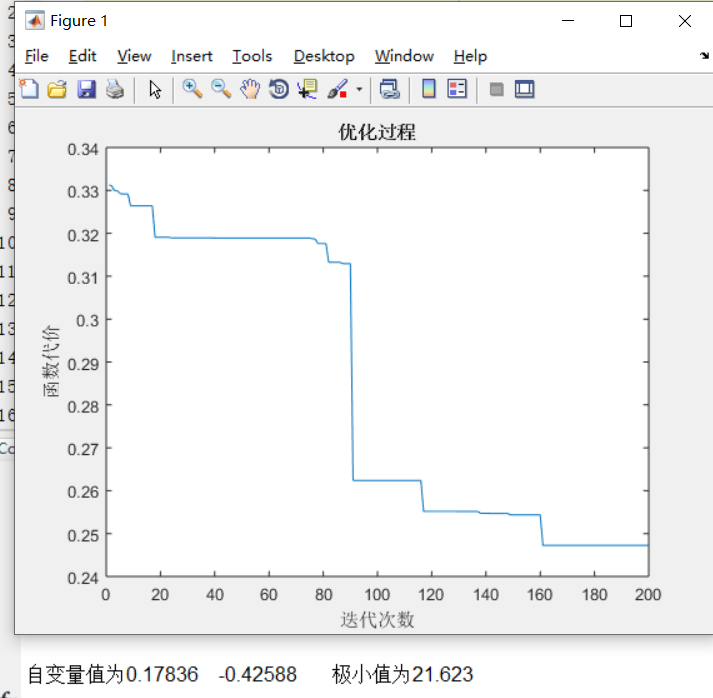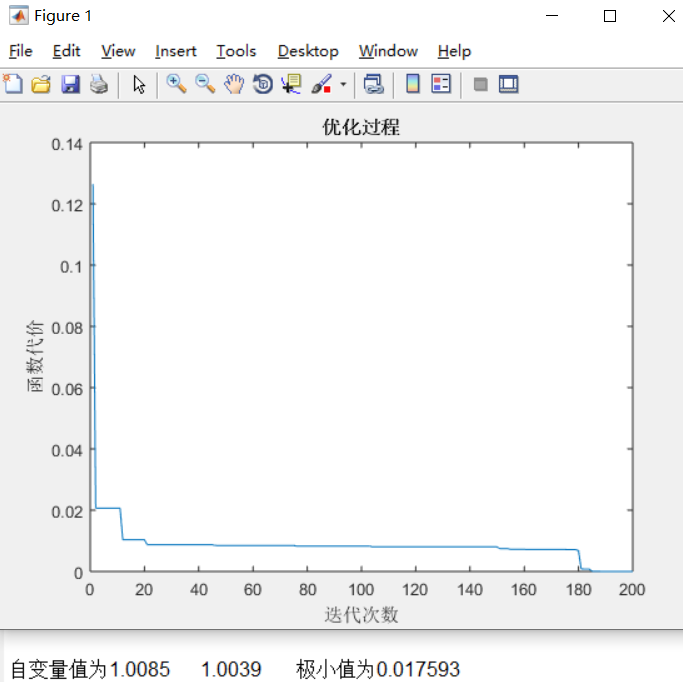从上图能够看到传统能够得到最终结果，但是稳定性较差，不易得到较好的结果
②对函数做平移，即可得到

其最小值位于时，最小值为0
多次运行结果为：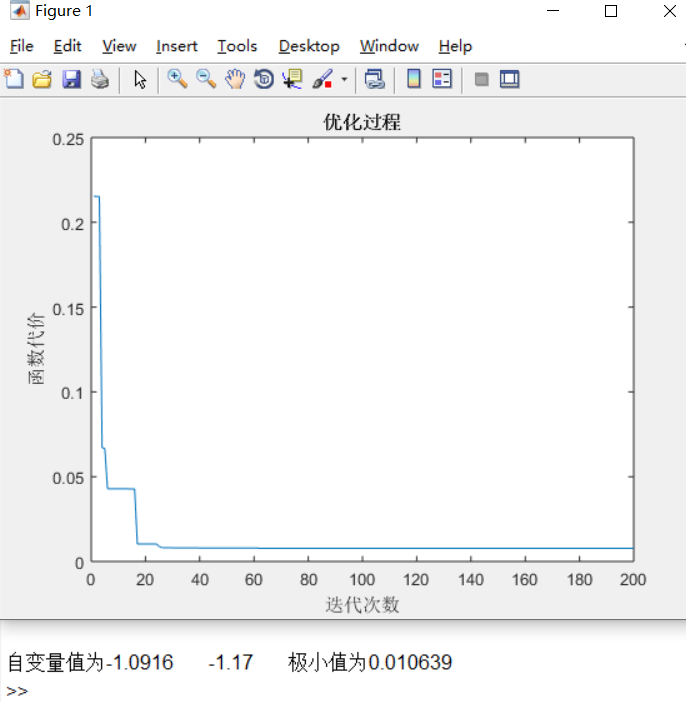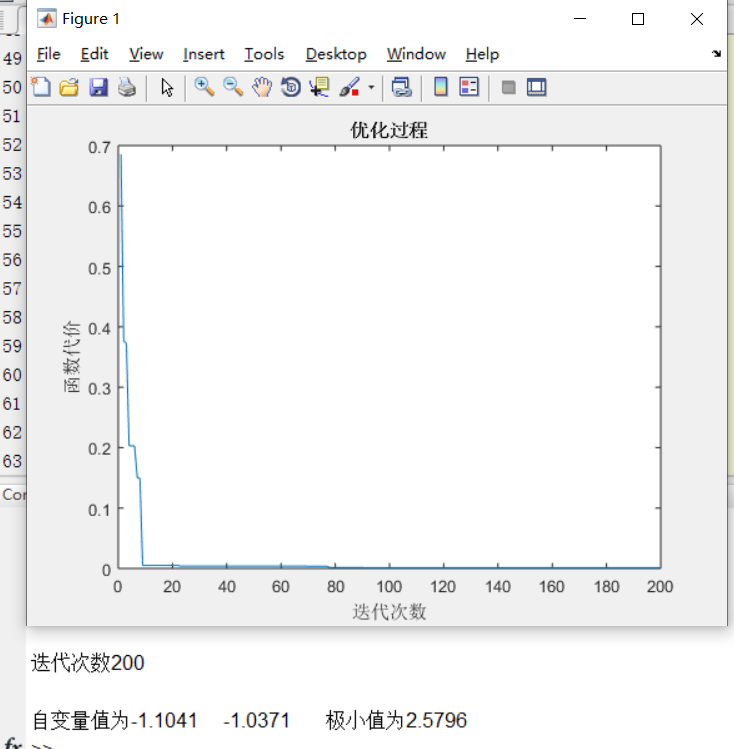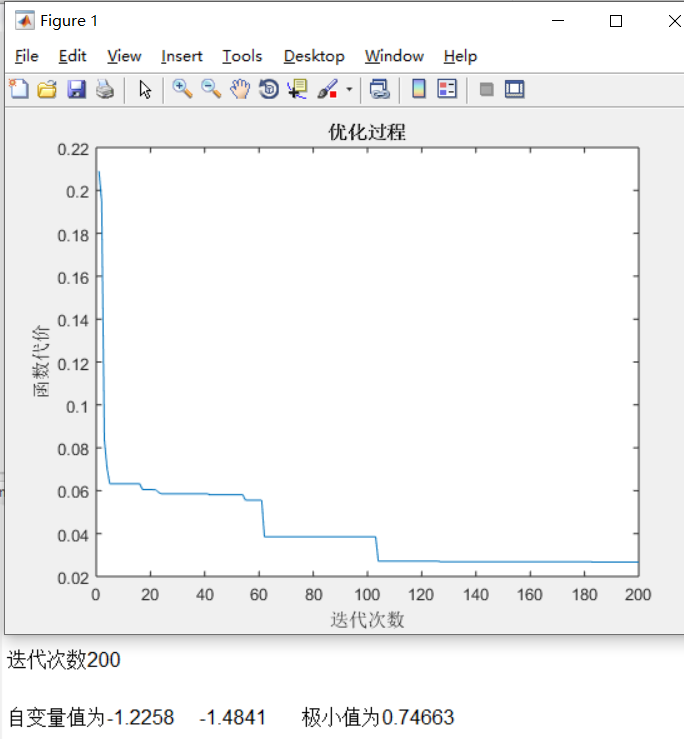结果与函数①类似
二、改进遗传算法求解函数最小值
同样求函数②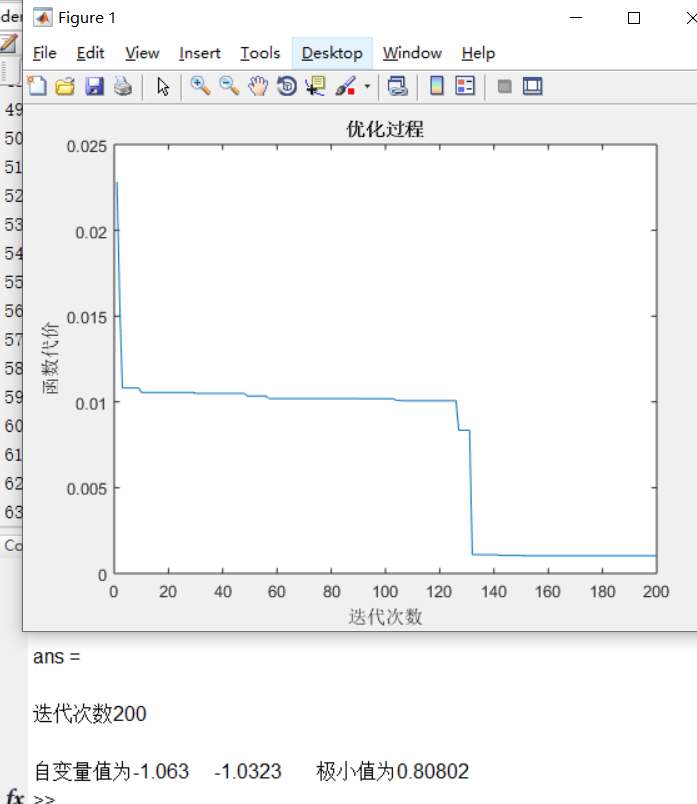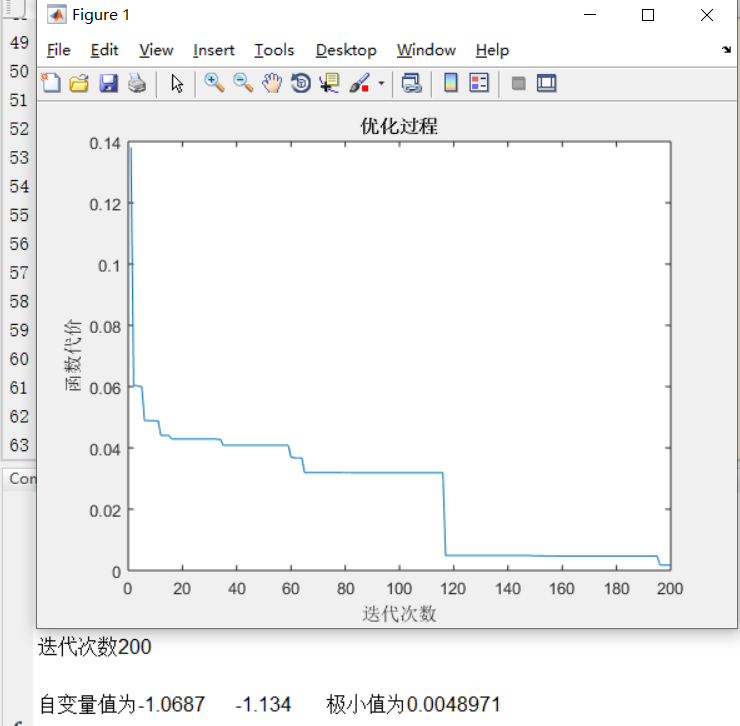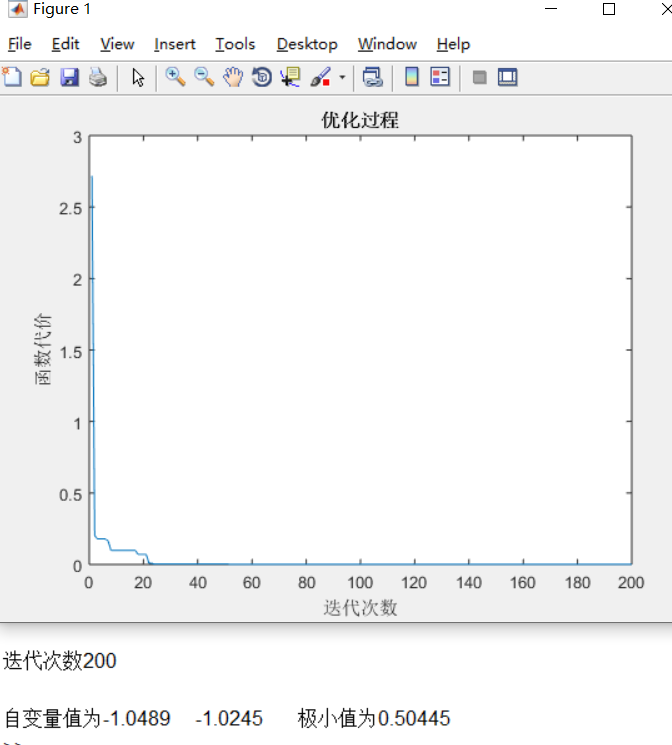能够看出改进后的结果更稳定，结果更接近实际最小值。
三、代码：https://download.csdn.net/download/qq_44152192/19132048
展开全文算法
• 它使用粒子群优化算法找到变量函数最小值。 % 输入参数为： % -func: 目标函数的最小化句柄％-numInd：这是群体元素的数量% -range: 必须创建元素的范围% -n_var: 函数变量的个数% -tolerance：它是群体停止标准...
• 求助Matlab关于三个自变量的函数最小值优化求解重发-需要解决的问题.doc 本人一直做解析理论物理推导的，可惜不幸的事情是，本人的数值计算功底很差，看到附件里面的三个自变量参数的最小值优化就呆了，带有三个...
• 能够求解规定定义域内函数的最值问题，包括负数域，同时进行了一定的改进，使遗传算法的计算更加稳定
• 梯度下降法是一个最优化算法，可以用来求一个函数最小值，最大值等，也常用于人工神经网络中更新各个感知器之间的权值，求出cost function的最小值等，应用广泛。  其原理简单，就求函数最小值这个应用而言，...
算法原理

梯度下降法是一个最优化算法，可以用来求一个函数的最小值，最大值等，也常用于人工神经网络中更新各个感知器之间的权值，求出cost function的最小值等，应用广泛。  其原理简单，就求函数的最小值这个应用而言，大致说来就是先求出该函数梯度，大家都知道梯度是一个函数在该点的最快上升率，那么求个相反数就是最快下降率了。将每个自变量按下列公式更新：  xt+1=xt+Δx xt+1=xt+Δx
其中  Δx=−η∂f∂x Δx=−η∂f∂x

ηη称为学习速率，也称为步长，指明了自变量朝向最快下降方向减少的速度，这个值的选定相当重要，太大了会导致震荡，无法收敛到最小值点，太小了会导致收敛速度过慢。  当xt+1xt+1和xtxt的差小于一个阀值时，就可以停止循环了。

Matlab实现
% 没有加入冲量项的随机梯度下降法实现
syms x y real
y(x) = x^2+2*x+10 ;
delta(x) = -diff(y(x)) ;
step = 0.1 ;
first_x = 10 ;
x_current = first_x ;
x_next = first_x ;
show_tmp = 0 ;
show = [] ;
counter = 0 ;
delta_last = 0 ;
while (true)
delta_x = double(delta(x_current))*step ;
if(abs(delta_x) < 0.0001)
break
end
x_next = x_current + delta_x ;
x_current = x_next ;
counter = counter + 1;
show_tmp = x_next ;
show = [show, show_tmp] ;
if(counter > 200)
break ;
end
end
x_next
counter
figure(1)
ezplot(y(x))
hold on
plot([-100, 100],[double(y(x_next)), double(y(x_next))],'-r')
double(y(x_next))
figure(2)
plot(show)
pause
close all

为了来定义我们预测值和真实值之间的差异，我们引入代价函数(cost function)的概念所以，现在我们的任务就是来求得一个Θ，从而使得代价函数达到最小，也即全局最小(global minimun).在这里我们使用梯度下降算法来求函数的最小值。

梯度下降法是按下面的流程进行的：
1）首先对θ赋值，这个值可以是随机的，也可以让θ是一个全零的向量。
2）改变θ的值，使得J(θ)按梯度下降的方向进行减少。

如下图：上图表示的是参数Θ和代价函数J(Θ)的关系图，深蓝色为全局最小，浅蓝色为局部最小，红色则表示J(Θ)有一个较大的取值，而梯度下降算法就是我们给定一个初始的Θ值，然后按照梯度下降的原则不断更新Θ值，使得J(Θ)向更低的方向进行移动。算法的结束将是在θ下降到无法继续下降为止。上面两条线代表我们给定两个初值，我们发现一条到达局部最小，即浅蓝色，而一条到达全局最小，即深蓝色。所以从这里我们可以看出，初始值的选择对梯度下降的影响很大。
梯度下降的更新规则：解释：描述：对 θ 赋值，使得 J(θ)按梯度下降最快方向进行，一直迭代下去，最终得到局部最小值。 其中 α 是学习率（ learning rate） ， 它决定了我们沿着能让代价函数下降程度最大的方向向下迈出的步子有多大。
对于梯度下降，我们再来直观的画出图像理解一下，J(Θ)的图像我们如下求导的目的，基本上可以说取这个红点的切线，就是这样一条红色的直线，刚好与函数相切于这一点，让我们看看这条红色直线的斜率，就是这条刚好与函数曲线相切的这条直线，这条直线的斜率正好是这个三角形的高度除以这个水平长度，现在，这条线有一个正斜率，也就是说它有正导数， 因此， 我得到的新的 θ1， θ1 更新后等于 θ1 减去一个正数乘以 α。当Θ取到最小，J(Θ)最小，也即Θ不能小时算法结束。

对于α ，后面会讲到，这里先简单提下：
如果 α 太小了，即我的学习速率太小，结果就是只能这样像小宝宝一样一点点地挪动，去努力接近最低点，这样就需要很多步才能到达最低点，所以如果 α 太小的话，可能会很慢因为它会一点点挪动，它会需要很多步才能到达全局最低点。 如果 α 太大，那么梯度下降法可能会越过最低点，甚至可能无法收敛， 下一次迭代又移动了一大步，越过一次，又越过一次，一次次越过最低点，直到你发现实际上离最低点越来越远，所以，如果 α 太大，它会导致无法收敛，甚至发散。

当只有一个训练样本时，执行梯度下降算法（注意这里只是对Θj求导，所以最后乘上的是xj）所以针对一个训练样本，更新规则如下：现在推广到存在多个特征时注意：迭代都是需要同时进行的。在进行随机梯度下降算法时，我们每次迭代都只选取一个训练样本，这样当我们迭代到若干样本的时候Θ就已经迭代到最优解了。

展开全文• 本文件使用粒子群算法计算出二元函数最小值，运行速度快。得到的最小值非常接近最优值。只需修改对应的函数表达式，根据所需要的区间，即可运行
• 差分进化算法求解平方和函数最小值，VS2013编程，C++语言C++
• 粒子群算法 求函数最小值matlab代码。链接是讲解粒子群算法 https://blog.csdn.net/zyqblog/article/details/80829043 。 我用ubuntu下matlab编写的代码，你在windows下可能有中文乱码，不过都是注释，你可以去...
• 这是一种计算函数最小值的简单遗传算法。 它没有任何改进，但我有一些像精英主义、代沟和重整化这样的改进。
• 将上述各函数值进行比较,最终确定出在 D 内的最大值和最小值。 3.函数求偏导数的 MATLAB 命令 MATLAB...线性规划问题是在一组线性约束条件的限制下,求一线性目标函数最大或 最小值的......MATLAB实验报告,遗传算法解...

将上述各函数值进行比较,最终确定出在 D 内的最大值和最小值。 3.函数求偏导数的 MATLAB 命令 MATLAB 中主要用 diff 求函数的偏导数,用 jacobian 求 ......
第七讲 Matlab 优化(求极值)理论介绍:算法介绍、软件求解. 一.线性规划问题 1.线性规划问题是在一组线性约束条件的限制下,求一线性目标函数最大或 最小值的......
MATLAB实验报告,遗传算法解最短路径以及函数最小值问题讲解_中职中专_职业教育_教育专区。MATLAB 硕士生考查课程考试试卷 考试科目: 考生姓名: 学院: MATLAB 教程 ......
Ma ab中求函数的最小值_数学_自然科学_专业资料 人阅读|次下载 Ma ab中求函数的最小值_数学_自然科学_专业资料。MATLAB 优化应用 §1 线性规划模型 一、......
将上述各函数值进行比较,最终确定出在 D 内的最大值和最小值。 3.函数求偏导数的 MATLAB 命令 - MATLAB 中主要用 diff 求函数的偏导数,用 jacobian 求 ......
将上述各函数值进行比较,最终确定出在 D 内的最大值和最小值。 3.函数求偏导数的 MATLAB 命令 MATLAB 中主要用 diff 求函数的偏导数,用 jacobian 求 ......
15、 平均值、标准方差 MATLAB 提供了 mean,std 函数来计算平均值、标准方差或方差。这些函数的调用方法如下: mean(x):返回向量 x 的算术平均值。 std(x):......
x1-x2 +x3 -3x4 =1 x1 -x2 -2x3 +3x4 =- 1 2 二.非线性一元函数的最小值 对于求一元函数的最小值问题,Matlab 提供了一个命令函数 fminbnd, ......
数据分析与多项式计算 6.1 数据统计处理 6.1.1 最大值和最小值 MATLAB 提供的求数据序列的最大值和最小值的函数分别为 min,两个函数的调用格式和操作过程......
作函数曲线: 作函数曲线 fplot(y, [-4,2]) 3.求函数在给定区间上的最值 3.求函数在给定区间上的最值求函数在给定区间上的最小值点的 MATLAB 命令是 ......
MATLAB 多元函数导数求极值或最优值 实验六 多元函数的极值 【实验目的】 1. 多元函数偏导数的求法。 2. 多元函数自由极值的求法 3. 多元函数条件极值的求法、 4. 学习掌握 MATLAB 软件有关的命令。 【实验内容】 求函数 z x......
x1-x2 +x3 -3x4 =1 x1 -x2 -2x3 +3x4 =- 1 2 二.非线性一元函数的最小值 对于求一元函数的最小值问题,Matlab 提供了一个命令函数 fminbnd, fminbnd 函数的调用格式为:X=fminbnd(fun,x1,x2)和[X,fval]=fminbnd......
要求设计遗传算法对该问题求解。 b e 1 2 h 2 6 3 9 7 a 8 1 6 2 k c f i 7 4 1 1 4 d 9 g 1 j B、设计遗传算法求解 f(x)极小值,具体表达式如下: 要求必须使用 m 函数方式设计程序。 C、利用 MATLAB ......
例 3.6.2 画出上例中函数的图形 解 syms x ↙ y=(3*x^2+4*x+4)/( x^2+x+1); ↙得到如下图形 ezplot(y) ↙↙ 如何用 MATLAB 求函数的极值点和最大值 比如说 y=x^3+x^2+1,怎样用 matlab 来算它的极值和最......
matlab自定义函数与极值求法_IT/计算机_专业资料。实验 5 matlab 自定义函数与导数应用实验目的1.学习 matlab 自定义函数. 2.加深理解罗必塔法则、极值、最值、单调性. 实验内容1.学习 matlab 自定义函数及求函数最小值 ......
第6 章 MATLAB 数据分析与多项式计算 6.1 数据统计处理 6.1.1 最大值和最小值 MATLAB 提供的求数据序列的最大值和最小值的函数分别为 max 和 min,两个函数的调用格式和操作过程类似。 1.求向量的最大值和最小值 求一个......
将上述各函数值进行比较,最终确定出在 D 内的最大值和最小值。 3.函数求偏导数的 MATLAB 命令 MATLAB 中主要用 diff 求函数的偏导数,用 jacobian 求 Jacobian 矩阵。 diff(f,x,n) 求函数 f 关于自变量 x 的 n 阶导数。 ...
将上述各函数值进行比较, 最终确定出在 D 内的 最大值和最小值。 3.函数求偏导数的 MATLAB 命令 MATLAB 中主要用 diff 求函数的偏导数,用 jacobian 求 Jacobian 矩阵。 diff(f,x,n) 求函数 f 关于自变量 x 的 n 阶导数。...
输入20个数,求其中最大数和最小数。要求分别用循环结构和调用MATLAB的max函数、min函数来实现。 li3eabc |2018-06-30 | 2.1分(高于23.30%的文档)|6653|43 | 举报 马上扫一扫 手机打开随时查看 手机打开 专业文档 专业文档是......
? ? ? ? 4. 数值函数的最小(大)值 求一元函数或多元函数的最小(大)值是数学上 经常遇到的问题,如何求解最小(大)值, MATLAB提供了相应的命令fminbnd(一元函 数最小值)和fminsearch(多元函数最小值)。 求一元函数f(x)在......

展开全文• 利用C++语言模拟遗传算法进行对Rosenbrocks函数最小值求值。之前自动涨上去的，重新把积分减下来了
• 一、函数的极小值和零点fminbnd(求单变量非线性的极小值)(局部最优)clear all;close all;[x,fval]=fminbnd(@cos,1,5) %返回一个值 x，该值是 ...要计算最小值函数必须是连续的。2.fminbnd 只能给出局部解。3.当解...


一、函数的极小值和零点
fminbnd(求单变量非线性的极小值)(局部最优)
clear all;
close all;
[x,fval]=fminbnd(@cos,1,5)    %返回一个值 x，该值是 fun 中描述的标量值函数在区间 1< x < 5 中的局部最小值
y=cos(x)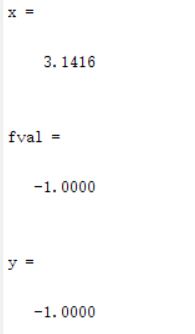该算法的局限性1.要计算最小值的函数必须是连续的。2.fminbnd 只能给出局部解。3.当解在区间的边界上时，fminbnd 可能表现出慢收敛。
二、求解自变量范围限定的一元函数的最小值
clear all;
close all;
f=@(x)x.^2-6*x-9;
[x,fval]=fminbnd(f,0,6,optimset('Display','iter'))
%自变量在0到6之间的最小值，显示计算过程
y=f(x)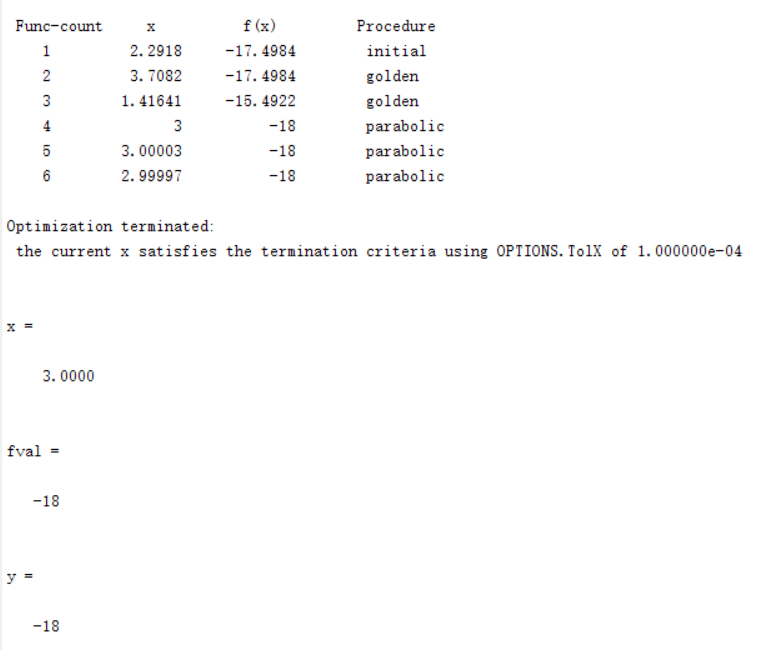三、求多元函数的最小值
fminsearch函数用来求解多维无约束的线性优化问题
clear all;
close all;
f=@(x)2*(x(2)-x(1)^2)^2+(2-x(1))^2;
[x,fval]=fminsearch(f,[-6.7 8])     %指定一个起始点  在某点附近寻找
y=f(x)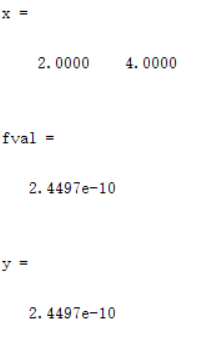四、求解一元函数的零点
fzero函数用来求单变量非线性方程的根
clear all;
close all;
f=@(x)sin(x)*cos(x);
figure; % 创建一个新的窗口，所有参数采用默认
fplot(f,[-5,5]); %绘制表达式或函数
xlabel('x');
ylabel('f(x)');
grid on; %打开网格
x1=fzero(f,[-4,-2])
x3=fzero(f,-2)
[x4,fval]=fzero(f,[-3,-1])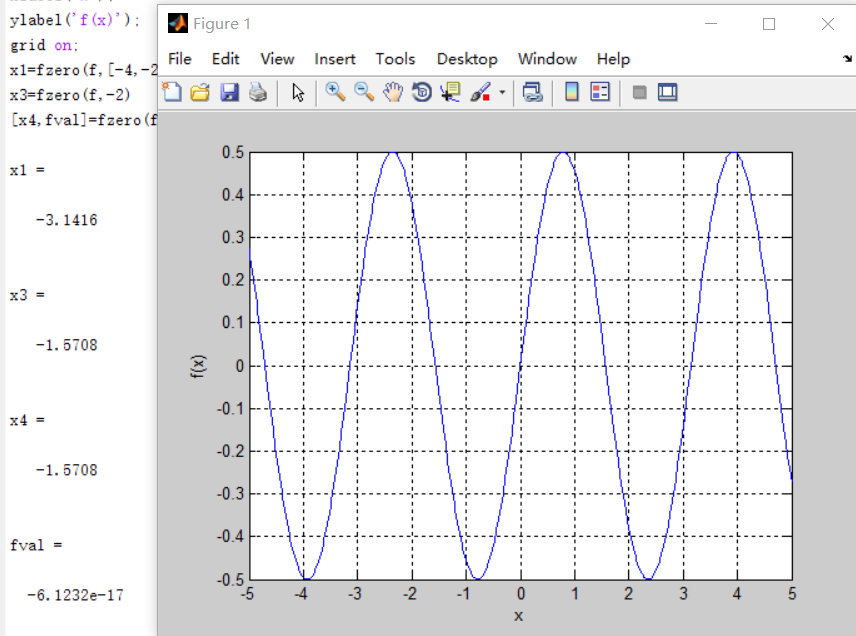展开全文• 今天小编就为大家分享一篇使用遗传算法求二元函数最小值，具有很好的参考价值，希望对大家有所帮助。一起跟随小编过来看看吧
• 最小值函数图像如图（及程序）所示： clear all; close all; clc; x=0:0.01:10; y=x+10*cos(5*x)+7*sin(4*x); figure plot(x,y) grid on xlabel('x') ylabel('f(x)') title('f(x)=x+10*cos(5*x)+7*sin(4*x)')...遗传算法
• %% 学习目标：求一元函数最小值和零点 %% 函数的极小值和零点 求解一元函数的最小值 clear all; close all; [x,fval]=fminbnd(@cos,1,5) %函数句柄 y=cos(x) %% 求解自变量范围限定的一元函数的最小值 clear all; ...
• 遗传算法用的是matlab实现的，用该算法求得是函数的极小值，并且程序都是底层程序没有用到顶层的工具包，能够更好的理解遗传算法的基本思想。遗传算法
• 主要介绍了python使用梯度下降和牛顿法寻找Rosenbrock函数最小值实例，具有很好的参考价值，希望对大家有所帮助。一起跟随小编过来看看吧
• 该实验使用模拟退火算法求取函数最小值matlab自编程实现。该实验可以观察到搜索点的过程，也可以自行修改参数。
• 利用智能算法之一的蚁群算法求最小值MATLAB实现蚁群算法
• 例 ： 求解函数最小值 粒子群算法的驱动因素 从鸟群觅食行为到粒子群算法 鸟群寻找食物的过程中，鸟与鸟之间存在着信息的交换，每只鸟搜索目前离食物最近的鸟的周围区域是找到食物的最简单有效的办法。 ...粒子群算法 粒子群更新公式 智能计算
• 牛顿法寻找函数最小值 %目标函数：f % 初始点：x0 % 精度：eps
• 4 附：CS算法求解函数最小值代码 5 源码下载 6 参考文献 1 从布谷鸟的育雏到布谷鸟算法 布谷鸟不会做窝，也不会育雏，在春末夏初，向北飞，趁别的鸟(宿主鸟)外出觅食时，将卵蛋产在宿主鸟窝里，让宿主鸟...莱维飞行 布谷鸟算法 CS算法 Levy
• 网络上免疫算法的java实现很少，因此本人花了几天时间自己写了eclipse平台下的基于函数最值求解的免疫算法，直接导入就可以使用，代码中参数设置还存在异议，可以联系我一起讨论！谢谢！java...

# matlab求解函数最小值matlab 订阅